# Grandmother's clocks

Grandmother's clock is late every hour by half a minute. Grandmother set the clock exactly at 8.00 am. How many hours will show after 24 hours?

Result

x = 7:48### Step-by-step explanation: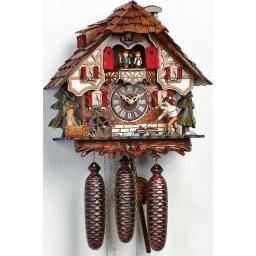Did you find an error or inaccuracy? Feel free to write us. Thank you!Tips to related online calculators
Need help to calculate sum, simplify or multiply fractions? Try our fraction calculator.
Check out our ratio calculator.
Do you want to convert time units like minutes to seconds?

## Related math problems and questions:

• Delayed clockMichael put a new battery into his watch at midnight. However, they are 5 seconds late each minute. How many hours does the watch show in 24 hours?
• A clockA clock was set right at 6:00 AM. If it gains 3 1/2 minutes per hour, what time will it show at 6:00 PM on the same day? Show your solution
• Quarter of an hourIf the clock showed a quarter of an hour more, it would be 10 minutes to 10 hours. How many hours do they show?
• Divers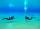20-member group of divers carries the air for 15 hours of diving. 4 members of the expedition sick or wasn't came. How many hours is sufficient air to other divers?
• HandsThe clock shows 12 hours. After how many minutes will angle between the hour and minute hand 90°? Consider the continuous movement of both hands hours.
• Magnitude of angleWhat magnitude has an obtuse angle enclosed by the hands of clocks at 12:20 hours?
• Clocks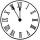What distance will describe the tip of minute hand 6 cm long for 20 minutes when we know the starting position with finally enclose hands each other 120°?
• Clock hands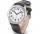The hands-on clock shows the time 12 hours and 2 minutes. Calculate the size of an acute angle between clock hands three hours later.
• ClocksHow will long path walk the end of the clock's minute-hand long 7 cm for 330 minutes? How long will walk in the same time hour-hand?
• Hour salaryYou work for 4 hours on a Saturday and 8 hours on Sunday. You also receive a $50 bonus. You earn$164. How much did you earn per hour?
• Five combers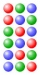Five combers harvest 12 rows of strawberries in 4 hours. How many rows of strawberries will two combers harvest in 10 hours?
• Two machinesThe first machine can produce parts in 24 hours, the second in 16 hours. The first started working at 6:00 pm. Second at 10:00 o'clock. At what hour were all parts made?
• Land area 2A land area was divided among the three heirs in the ratio 5:2:4. If the largest share was 20 hectares of land, what is the total area of land? Please show your solution and what kind of proportion is this please
• Harvesters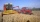The first harvester will harvest the grain from the field in 20 hours, the second, the more powerful in 10 hours. How long will it take to harvest this field by both harvesters if the second harvester has to be set up first and this takes 2 hours? The fir
• Jan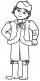Jan is 13 years and Peter 21 years old. For how many years will their ages in the ratio of 7:9?
• Forestry workersIn the forest is employed 56 laborers planting trees in nurseries. For 8 hour work day would end job in 37 days. After 16 days, 9 laborers go forth? How many days are needed to complete planting trees in nurseries by others if they work 10 hours a day?
• What time is it?What time is it, when there is four times less time left until midnight than the time that has elapsed since noon? What time is it, when one-fifth of the hours that have passed since midnight is equal to one-third of the hours that are missing by 12 o'clo